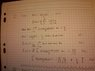# Fourier Mistake: Help Jenny Out

• jennyjones

#### jennyjones

can someone spot my mistake, I'm stuck

thanks,

jenny

#### Attachments

•fourier.jpg
32.6 KB · Views: 355
I don't think step 3 is correct. the original function is not zero for |x| > a. But it looks like you assume that so that you can make the integral over this range, instead of from minus infinity to infinity. At least, that looks like what you had in mind...

•1 person
Also, what definition are you using for the Fourier transform? Because I get the feeling they have used a different convention.

•1 person
When ##\lvert\omega\rvert < a##, you have
$$\int_0^\infty \frac{\sin ax\cos \omega x}{x}\,dx = \frac{\pi}{2}.$$ When ##\lvert\omega\rvert > a##, you have
$$\int_0^\infty \frac{\sin ax\cos \omega x}{x}\,dx = 0.$$ Somehow, you have to figure out what happens when ##\lvert\omega\rvert = a##.

•1 person
Seems like it might, like the Fourier series does at discontinuities. Do you know of a theorem that establishes the same result for the Fourier transform?

•1 person
I don't think step 3 is correct. the original function is not zero for |x| > a. But it looks like you assume that so that you can make the integral over this range, instead of from minus infinity to infinity. At least, that looks like what you had in mind...
Ah whoops, ignore this. I thought you wrote an ##a## for the upper limit, but it is an ##\infty##, as it should be.

jennyjones said:
bruce W I'm using the cosine transform, i made a picture of this formula for my textbook.
right. yes, you are using the same definition of the Fourier transform as they are. Which is good :)

jennyjones said:
Vela, do you know if i can than say |ω|= (∏/2+0)/2=∏/4 ?

the average?
I think you meant to say the average of the values of the Fourier transform on either side of the point ##\omega=a##. If this is what you meant, then yes that's right. Was it a guess? You have good intuition if it was. Yeah, there is a specific theorem (which is pretty hard to find on the internet), as vela is hinting at. This theorem works for certain kinds of function, like the rectangular function.

Yey! thank you, than i solve the problem now!

Do you maybe know the name of this theorem?

jenny

No worries :) I think wikipedia said it is a form of the Fourier inversion theorem. They didn't give a direct link for it though. It's on the wikipedia page "fourier inversion theorem", about halfway down under the subtitle "piecewise smooth; one dimension", if you are interested. It looks like the book "Fourier Analysis and its Applications" by Folland, G. B. might have some more information.